Importance: Medium ✭✭
 Author(s): Cameron, Peter J. Praeger, Cheryl E. Wormald, Nicholas C.
 Subject: Graph Theory » Infinite Graphs
 Keywords: arc transitive digraph infinite graph
 Posted by: mdevos on: October 29th, 2007
Conjecture   Ifis a highly arc transitive digraph with two ends, then every tile ofis a disjoint union of complete bipartite graphs.

It follows from a theorem of Dunwoody [D] that every vertex transitive graphwith two ends has a system of imprimitivitywith finite blocks so that the cyclic orderis preserved by the automorphism group (of). Ifis edge-transitive, then every edge ofmust have its ends in two consecutive blocks, so in this caseis an edge-disjoint union of the (isomorphic) bipartite graphsfor- which we shall call tiles. Note that the tiles are edge-transitive.

This gives us a good description of edge-transitive graphs with two ends; each is made up by gluing together copies of a tile in a linear order. Ifis a 2-arc transitive digraph with two ends, then all edges in each tile must be oriented consistently, so by possibly reordering, we may assume that every edge inis oriented fromto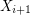. The above conjecture asserts that under the added symmetry condition of high arc transitivity, each tile has a simple structure - namely it is a union of (consistently oriented) complete bipartite graphs.

It is easy to construct a highly arc transitive two ended graph by simply using the complete bipartite graph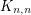(with all edges oriented consistently) as a tile. Mckay and Praeger found the following pretty construction of a highly arc transitive digraph with tiles isomorphic to a disjoint union of complete bipartite graphs: Letbe a finite set, letbe a positive integer, and defineto be the digraph with vertex setand an edge from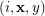to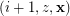if,, and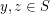. A generalized (twisted) version of this construction was introduced by Cameron, Praeger, and Wormald [CPW], but again, every tile in this construction is a disjoint unions of bipartite graphs, and it looks hard to do anything else.

## Bibliography

*[CPW] P. J. Cameron, C. E. Praeger, and N. C. Wormald, Infinite highly arc transitive digraphs and universal covering digraphs. Combinatorica 13 (1993), no. 4, 377--396. MathSciNet.

[D] M. J. Dunwoody, Cutting up graphs. Combinatorica 2 (1982), no. 1, 15--23. MathSciNet

* indicates original appearance(s) of problem.